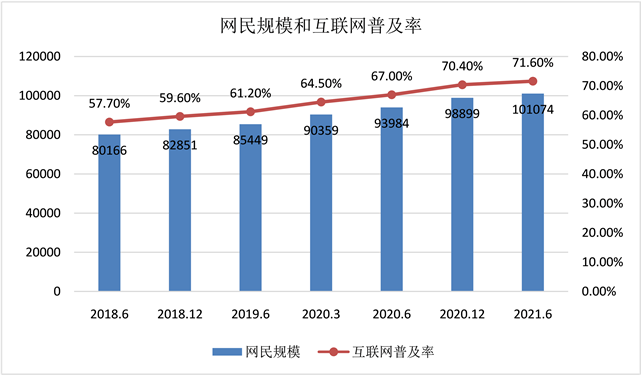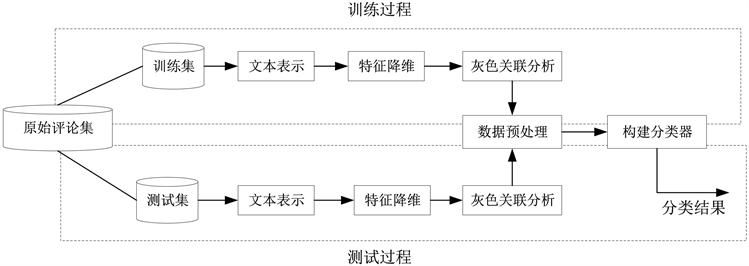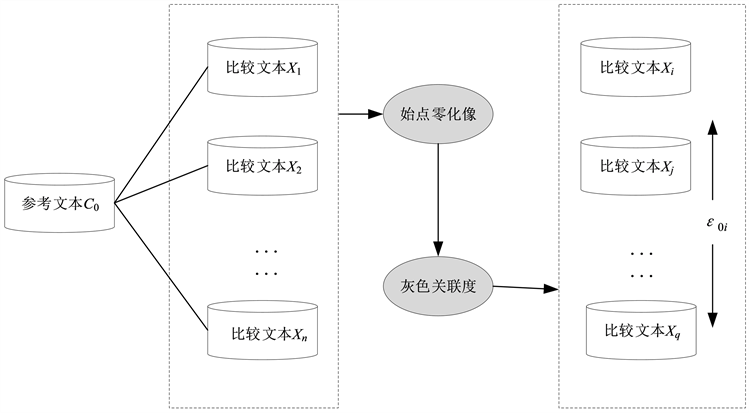#### 期刊菜单

Research on Online Review Classification Based on Improved Sentiment Analysis

Abstract: In the face of a large number of comments, it is cumbersome and inefficient to classify them only by relying on human resources. This paper proposes an improved online comment classification model based on sentiment analysis, which combines grey correlation analysis with Naive Bayes algorithm to consider the user sentiment orientation in online comments, and embed the result of grey correlation analysis as a feature attribute into the Naive Bayes classification model. This paper takes the Dyson V10 Fluffy Extra handheld wireless vacuum cleaner of JD.com as the research object, and mines real online review data to test the classification effect of the model. The results show that the classification performance of the improved model is significantly better than that of the traditional Naive Bayes classification model, and the evaluation index F value increases by 3.06%. This method has certain advantages in the application of online review text classification.

1. 引言数据来源：第48次《中国互联网络发展状况统计报告》

Figure 1. Internet user scale and Internet penetration rate

B2B电商规模整体扩大，口碑影响力日益凸显。随着社交媒体的发展，电子商务也逐渐形成了社会商务的新发展模式，在线用户评论也促进了电子商务发展 。消费者在电子商务平台扮演着非常重要的角色，在线评论引发的口碑传播形成的社会舆论效应影响着市场决策方向 。通过文本挖掘技术对在线评论文本提取有价值的信息，分析消费者对产品各个特征属性的评价及个人情感，成为电子商务网站研究的重点 。

2. 文本分类研究现状

2.1. 基于机器学习的情感分类方法

2.2. 基于朴素贝叶斯的分类算法

3. 模型构建Figure 2. Review classification framework

3.1. 文本表示

3.2. 特征降维

$IDF\left({t}_{k}\right)=\mathrm{log}\left(\frac{N}{n\left({t}_{k}\right)}+0.01\right)$ (1)

${W}_{k}=TF\left({t}_{k}\right)×IDF\left({t}_{k}\right)=\frac{TF\left({t}_{k}\right)×IDF\left({t}_{k}\right)}{\sqrt{{\sum }_{i=1}^{m}{\left(TF\left({t}_{k}\right)×IDF\left({t}_{k}\right)\right)}^{2}}}$ (2)

3.3. 灰色关联分析

1) 设情感词 ${w}_{ip}$ 的情感值为 ${P}_{{w}_{ip}}$，当 ${w}_{ip}$ 为正向情感词时，令 ${P}_{{w}_{ip}}=1$ ；当 ${w}_{ip}$ 为负向情感词时，令 ${P}_{{w}_{ip}}=-1$

2) 程度副词 ${w}_{iq}$ 的权重用 ${P}_{{w}_{iq}}$ 表示，当情感词 ${w}_{ip}$ 前面有程度副词 ${w}_{iq}$ 时，此时需要更新情感值，用 ${P}^{\prime }$ 表示：

${P}^{\prime }={P}_{{w}_{ip}}×{P}_{{w}_{iq}}$ (3)

3) 否定词 ${w}_{ir}$ 的权重用 ${P}_{{w}_{ir}}$ 表示，当情感词 ${w}_{ip}$ 前面有否定词 ${w}_{ir}$ 时， ${P}_{{w}_{ir}}=-1$，此时更新情感值，用 ${P}^{″}$ 表示：

${P}^{″}={P}_{{w}_{ip}}×{P}_{{w}_{ir}}$ (4)

4) 情感词 ${w}_{ip}$ 的最终情感值用 ${P}_{{w}_{ip}}$ 表示， ${P}_{{w}_{ip}}={P}^{\prime }+{P}^{″}$。将评论文本 ${d}_{i}$ 中所有情感值相加，得到该条评论的总体情感值 ${P}_{{d}_{i}}$

${P}_{{d}_{i}}=\underset{p}{\sum }{P}_{{w}_{ip}}$ (5)Figure 3. Grey correlation analysis

1) 构建参考序列和比较序列。由于存在n条评论，m维特征向量，因此可以假设 ${C}_{0}=\left({c}_{0}\left(1\right),{c}_{0}\left(2\right),\cdots ,{c}_{0}\left(m\right)\right)$ 代表参考序列，而 ${X}_{i}=\left({x}_{i}\left(1\right),{x}_{i}\left(2\right),\cdots ,{x}_{i}\left(m\right)\right),\left(i=1,2,\cdots ,n\right)$ 代表比较序列。

2) 求 ${C}_{\text{0}}$${X}_{i}$ 的始点零化像，记为 ${C}_{0}^{0}$ and ${X}_{i}^{0}$。由于不同序列的量纲不同，不可直接比较，因此需要将参考序列和比较序列的值转化为无量纲值。通过公式分别得到处理后的序列：

${C}_{0}^{0}=\left({c}_{0}^{0}\left(1\right),{c}_{0}^{0}\left(2\right),\cdots ,{c}_{0}^{0}\left(m\right)\right)$ (6)

${X}_{i}^{0}=\left({x}_{i}^{0}\left(1\right),{x}_{i}^{0}\left(2\right),\cdots ,{x}_{i}^{0}\left(m\right)\right),\left(i=1,2,\cdots ,n\right)$ (7)

${c}_{0}^{0}\left(k\right)={c}_{0}\left(k\right)-{c}_{0}\left(1\right),k=1,2,\cdots ,m$ (8)

${x}_{i}^{0}\left(k\right)={x}_{i}\left(k\right)-{x}_{i}\left(1\right),k=1,2,\cdots ,m;i=1,2,\cdots ,n$ (9)

3) 计算参考序列 ${C}_{\text{0}}$ 和比较序列 ${X}_{i}$ 的灰色绝对关联度 ${\epsilon }_{0i}$。本文使用公式

${\epsilon }_{0i}=\frac{1+|{{S}^{\prime }}_{0}|+|{{S}^{\prime }}_{i}|}{1+|{{S}^{\prime }}_{0}|+|{{S}^{\prime }}_{i}|+|{{S}^{\prime }}_{i}-{{S}^{\prime }}_{0}|},i=1,2,\cdots ,n$ (10)

$|{{S}^{\prime }}_{0}|=|\underset{k=2}{\overset{m-1}{\sum }}{c}_{0}^{0}\left(k\right)+\frac{1}{2}{c}_{0}^{0}\left(m\right)|$ (11)

$|{{S}^{\prime }}_{i}|=|\underset{k=2}{\overset{m-1}{\sum }}{x}_{i}^{0}\left(k\right)+\frac{1}{2}{x}_{i}^{0}\left(m\right)|,i=1,2,\cdots ,n$ (12)

$|{{S}^{\prime }}_{i}-{{S}^{\prime }}_{0}|=|\underset{k=2}{\overset{m-1}{\sum }}\left({x}_{i}^{0}\left(k\right)-{c}_{0}^{0}\left(k\right)\right)+\frac{1}{2}\left({x}_{i}^{0}\left(m\right)-{c}_{0}^{0}\left(m\right)\right)|,i=1,2,\cdots ,n$ (13)

3.4. 朴素贝叶斯文本分类器改进

$P\left({C}_{j}\right)=\frac{|{C}_{j}|}{|{T}_{train}|}$ (14)

$P\left({d}_{i}|{C}_{j}\right)=P\left(\left\{{t}_{1},{t}_{2},\cdots ,{t}_{m}\right\}|{C}_{j}\right)=\underset{k=1}{\overset{m}{\prod }}P\left({t}_{k}|{C}_{j}\right)$ (15)

$P\left({C}_{j}|{d}_{i}\right)=\frac{P\left({d}_{i}|{C}_{j}\right)P\left({C}_{j}\right)}{P\left({d}_{i}\right)}$ (16)

${C}_{map}=\underset{{C}_{j}\in C}{\mathrm{max}}P\left({C}_{j}|{d}_{i}\right)=\underset{{C}_{j}\in C}{\mathrm{max}}P\left({d}_{i}|{C}_{j}\right)P\left({C}_{j}\right)=\underset{{C}_{j}\in C}{\mathrm{max}}P\left(\left\{{t}_{1},{t}_{2},\cdots ,{t}_{m}\right\}|{C}_{j}\right)P\left({C}_{j}\right)$ (17)

4. 实证分析

4.1. 在线评论数据采集Table 1. Online comment collection

4.2. 情感分析结果Table 2. Identification results of “feature-point”

4.3. 分类结果与对比

1) 评价指标

F-measure是用来衡量分类器的综合性能的指标。AUC表示ROC曲线所覆盖的区域面积值，AUC的取值范围在0.5到1之间。AUC可以直观地评价分类器分类性能的好坏，值越大表示分类性能越好。

F-measure： $\text{F-measure}=\frac{\text{2}\cdot \text{Precision}\cdot \text{Recall}}{\text{Precision}+\text{Recall}}$ (20)

2) 模型分类结果Table 3. Model comparison results

5. 结语

1) 解决了研究数据的规范问题。在预处理部分，采用准确的方法实现文本分词、去除停用词、特征降维等工作。选取京东商城用户在线评论真实数据进行了实例验证，结果显示形成的数据集是规范可用的。

2) 提高了朴素贝叶斯文本分类器的分类性能。文章考虑在线评论中的用户情感倾向，将灰色关联分析结果作为一项特征属性嵌入朴素贝叶斯文本分类模型中。结果显示，改进后的模型的分类性能明显领先于传统朴素贝叶斯分类模型，评价指标F值提升了3.06%，表明该方法在在线评论文本分类应用中具有一定的优势。

  佚名. 第48次《中国互联网络发展状况统计报告》发布超十亿用户接入互联网我国成全球最庞大数字社会[J]. 网络传播, 2021(9): 76-81.  祝琳琳, 李贺, 李世钰, 等. 面向电子商务网站的在线评论信息质量感知机理研究[J]. 图书情报工作, 2021, 65(6): 32-40.  谢法举, 刘臣, 唐莉. 在线评论情感分析研究综述[J]. 软件导刊, 2018, 17(2): 1-4+7.  赵宇晴, 阮平南, 刘晓燕, 等. 基于在线评论的用户满意度评价研究[J]. 管理评论, 2020, 32(3): 179-189.  Arndt, J. (1967) Role of Product-Related Conversations in the Diffusion of a New Product. Journal of Marketing Research, 4, 291-295. https://doi.org/10.1177/002224376700400308  Chatterjee, P. (2001) Online Reviews: Do Consumers Use Them? Advances in Consumer Research, 28, 129-133.  李磊, 宋建伟, 刘继. 基于在线评论情感分析的声誉影响效应研究[J]. 管理学报, 2020, 17(4): 583-591.  何有世, 王明. 基于多特征组合的在线产品评论情感挖掘研究[J]. 软件导刊, 2017, 16(5): 1-5.  Das, S.R. and Chen, M.Y. (2007) Yahoo! for Amazon: Sentiment Extraction from Small Talk on the Web. Management Science, 53, 1375-1388. https://doi.org/10.1287/mnsc.1070.0704  Georgiadou, E., Angelopoulos, S. and Drake, H. (2020) Big Data Analytics and International Negotiations: Sentiment Analysis of Brexit Negotiating Outcomes. International Journal of Information Management, 51, Article ID: 102048. https://doi.org/10.1016/j.ijinfomgt.2019.102048  Whissell, C. (2009) Using the Revised Dictionary of Affect in Language to Quantify the Emotional Undertones of Samples of Natural Language. Psychological Reports, 105, 509-521. https://doi.org/10.2466/PR0.105.2.509-521  Wang, L., Guo, W., Yao, X., et al. (2021) Multimodal Event-Aware Network for Sentiment Analysis in Tourism. IEEE MultiMedia, 28, 49-58. https://doi.org/10.1109/MMUL.2021.3079195  Sanglerdsinlapachai, N., Plangprasopchok, A., Tu, B.H., et al. (2021) Improving Sentiment Analysis on Clinical Narratives by Exploiting UMLS Semantic Types. Artificial Intelligence in Medicine, 113, Article ID: 102033. https://doi.org/10.1016/j.artmed.2021.102033  Zhang, K., Zhu, Y., Zhang, W., et al. (2021) Cross-Modal Image Sentiment Analysis via Deep Correlation of Textual Semantic. Knowledge-Based Systems, 216, Article ID: 106803. https://doi.org/10.1016/j.knosys.2021.106803  贾晓帆, 何利力. 融合朴素贝叶斯与决策树的用户评论分类算法[J]. 软件导刊, 2021, 20(7): 1-5.  Kollur, J. and Razia, S. (2020) Text Classification Using Nave Bayes Classifier. Materials Today: Proceedings, in Press. https://doi.org/10.1016/j.matpr.2020.10.058  Hindi, K., Alijulaidan, R.R. and Alsalman, H. (2020) Lazy Fine-Tuning Algorithms for Nave Bayesian Text Classification. Applied Soft Computing, 96, Article ID: 106652. https://doi.org/10.1016/j.asoc.2020.106652  Wei, Z. and Feng, G. (2011) An Improvement to Naive Bayes for Text Classification. Procedia Engineering, 15, 2160-2164. https://doi.org/10.1016/j.proeng.2011.08.404  Zhang, H., Jiang, L. and Yu, L. (2021) Attribute and Instance Weighted Naive Bayes. Pattern Recognition, 111, Article ID: 107674. https://doi.org/10.1016/j.patcog.2020.107674  熊志斌, 刘冬. 朴素贝叶斯在文本分类中的应用[J]. 软件导刊, 2013, 12(2): 49-51.